# Three Dimensional Figures

In the Geometry section of GRE quantitative reasoning syllabus, three-dimensional figures hold an important place. And every GRE aspirant should be certain that they would encounter and solve at least one or two questions based on this topic in the exam. Three-dimensional figures even though seem intimidating needs just a bit of effort to master it. So, don’t neglect it, in place master it to score well on D- day.

So, what is a three- dimensional figure?

A three-dimensional figure always has length, breadth, and height whereas; a two-dimensional will only have length and breadth. Common three dimensional figures to appear in the syllabus of GRE are cube, cuboid, prism, cone etc.

Let us understand these shapes one at a time along with their volume and surface area formulas.

Cube: It is a square box whose all sides are equal and spread at an angle of 90°.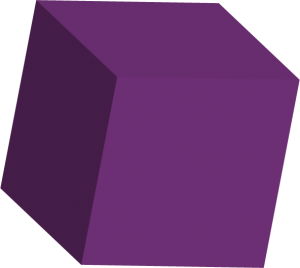$Volume \; of \; Cube = a \times a \times a = a^{3}$

Surface Area of Cube $= 2 \times length \times height + 2 \times base \times length + 2 \times base \times height = 6a^{2}$

Where, a = base = length = height and a = side of the cube

Cuboid: Cuboid is a rectangular solid.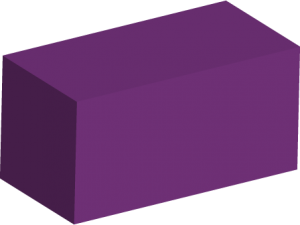$Volume \; of \; Cuboid = Length \times Breadth \times Height$

Surface Area of Cuboid =

Surface Area of Cuboid $= 2 \times length \times height + 2 \times base \times length + 2 \times base \times height = 2(lh + bl + bh)$

Sphere: Balls, football, cricket ball etc. are example of spheres.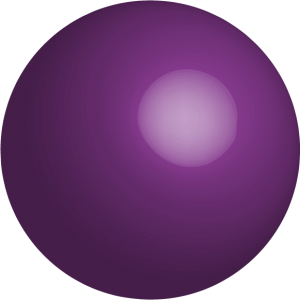$Volume \; of \; Sphere = V = \frac{4}{3} \pi r^{2}$ $Surface \; Area \; of \; Sphere = A = 4 \pi r^{2}$

Pyramid: You can even calculate the volume and surface area of the great Pyramids of Egypt. Shocked? Don’t be! The Great Pyramids of Egypt are example of the pyramids that we study in three- dimensional geometry.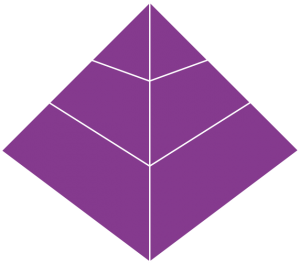$Volume \; of \; Pyramid = \frac{1}{3} \times Area \; of \; base \times height = \frac{1}{3} \times A \times h$ $Surface \; Area \; of \; Pyramid = l \times b + l \sqrt{\left ( \frac{b}{2} \right )^{2} + h^{2}} + b \sqrt{\left ( \frac{l}{2} \right )^{2} + h^{2}}$

Where, l = length, b = breadth, h = height

Square Pyramid: Square pyramid is a type of pyramid that has square base. It is a polyhedron in nature.$Volume \; of \; a \; Square \; Pyramid = (side \; of \; square)^{2} \times \frac{height}{3}$ $Surface \; Area \; of \; a \; Square \; Pyramid = a^{2} + 2a \sqrt \frac{a^{2}}{4} + h^{2}$

Cone: Ever enjoyed the delicious and chilling ice-cream cone? Who hasn’t! Ice- creams are favorites of all but have you ever paid attention to the shape of the cone? It is circular at one side and pointed at the other.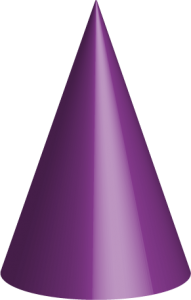$Volume \; of \; a \; Cone = V = \pi r^{2} \frac{h}{3}$$Surface \; Area \; of \; a \; Cone = A = \pi r (r + \sqrt{h^{2} + r^{2}})$ $Surface \; Area \; of \; a \; Cone = A = \pi r (r + \sqrt{h^{2} + r^{2}})$

Cylinder: Cylinder is a solid geometrical figure with straight parallel sides and a circular or oval cross section.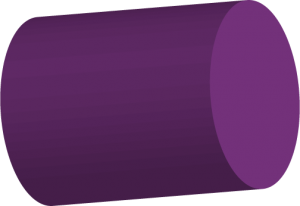$Volume \; of \; a \; Cylinder = V = \pi r^{2}h$ $Surface \; Area \; of \; a \; Cylinder = A = 2\pi rh + 2 \pi r^{2}$

BYJU’S will be glad to help you in your GRE preparation journey. You can ask for any assistance related to GRE from us by just giving a missed call at +918884544444, or you can drop an SMS. You can write to us at gre@byjus.com.